Instantly share code, notes, and snippets.

# endolith/A_weighting.py

Last active December 13, 2022 04:50
A-weighting audio files in Python
This file contains bidirectional Unicode text that may be interpreted or compiled differently than what appears below. To review, open the file in an editor that reveals hidden Unicode characters. Learn more about bidirectional Unicode characters
 #!/usr/bin/env python # -*- coding: utf-8 -*- """ Translated from a MATLAB script (which also includes C-weighting, octave and one-third-octave digital filters). Author: Christophe Couvreur, Faculte Polytechnique de Mons (Belgium) couvreur@thor.fpms.ac.be Last modification: Aug. 20, 1997, 10:00am. BSD license http://www.mathworks.com/matlabcentral/fileexchange/69 Translated from adsgn.m to Python 2009-07-14 endolith@gmail.com """ from numpy import pi, polymul from scipy.signal import bilinear def A_weighting(fs): """Design of an A-weighting filter. b, a = A_weighting(fs) designs a digital A-weighting filter for sampling frequency `fs`. Usage: y = scipy.signal.lfilter(b, a, x). Warning: `fs` should normally be higher than 20 kHz. For example, fs = 48000 yields a class 1-compliant filter. References:  IEC/CD 1672: Electroacoustics-Sound Level Meters, Nov. 1996. """ # Definition of analog A-weighting filter according to IEC/CD 1672. f1 = 20.598997 f2 = 107.65265 f3 = 737.86223 f4 = 12194.217 A1000 = 1.9997 NUMs = [(2*pi * f4)**2 * (10**(A1000/20)), 0, 0, 0, 0] DENs = polymul([1, 4*pi * f4, (2*pi * f4)**2], [1, 4*pi * f1, (2*pi * f1)**2]) DENs = polymul(polymul(DENs, [1, 2*pi * f3]), [1, 2*pi * f2]) # Use the bilinear transformation to get the digital filter. # (Octave, MATLAB, and PyLab disagree about Fs vs 1/Fs) return bilinear(NUMs, DENs, fs)
This file contains bidirectional Unicode text that may be interpreted or compiled differently than what appears below. To review, open the file in an editor that reveals hidden Unicode characters. Learn more about bidirectional Unicode characters
 import sys from scipy.signal import lfilter import numpy as np from A_weighting import A_weighting try: import soundfile as sf except ImportError: from scikits.audiolab import wavread # (scipy.io.wavfile can't read 24-bit WAV) def rms_flat(a): # from matplotlib.mlab """ Return the root mean square of all the elements of *a*, flattened out. """ return np.sqrt(np.mean(np.absolute(a)**2)) if len(sys.argv) == 2: filename = sys.argv else: filename = 'noise.wav' try: x, fs = sf.read(filename) except NameError: x, fs, bits = wavread(filename) print(filename) print('Original: {:+.2f} dB'.format(20*np.log10(rms_flat(x)))) b, a = A_weighting(fs) y = lfilter(b, a, x) print('A-weighted: {:+.2f} dB'.format(20*np.log10(rms_flat(y))))

### Yan-Iron commented Aug 2, 2018

Dear all, I am new to digital signal processing. I am wondering, in order to get A weighted sound pressure level, should I first apply this function to the wav data read by functions like "scipy.io.wavfile.read" then convert it to SPL, or first convert wav data to sound pressure level then use this function for A weighting? Thanks in advance!

### endolith commented Aug 2, 2018

@shangyan0517 I would recommend:

Then you need to figure out what your dBSPL dBFS conversion factor is. Depends on sensitivity of microphone, gain of preamp, etc.

It doesn't matter if you do SPL conversion first, then A-weighting, or A-weighting, then SPL conversion. Both are LTI operations.

### Yan-Iron commented Aug 3, 2018

@endolith Thank you so much!

### masahf commented Aug 21, 2020

@endolith Thank you for the helpful code.
I am new to digital signal processing.
I'm doing a project with digital MEMS microphones and using audiobusio library that has PDMin for recording over i2s with CircuitPython. My goal is to convert the digital output signal into dB SPL but still don't know how to do it :( Can I use this function for the I2S output signal?

### annezhangxue commented Jan 24, 2021

@endolith, Thank you for the code, it works out perfectly!
I am also new in acoustics, could you please let me know if this is based on octave band or one third octave band please?

Thanks.

### endolith commented Jan 25, 2021

@annezhangxue It is not based on octave bands. 🤔 It's an IIR filter

### ThomQu commented Oct 8, 2021 • edited

@endolith

Hi!

I've been testing your code and Idon't fully get it: It doesn't weight the signal at high frequencies. here's a fig with:

• the A-weights from IEC 1672 norm (blue);
• the polynom NUMs/DENs from your function (green);
• the difference between the spectrum of an A-weigted white noise signal, and the same signal without A weighting.

As you can see, the polynom tends to zero for high frequencies, causing the curve 3 to diverge from the expected result...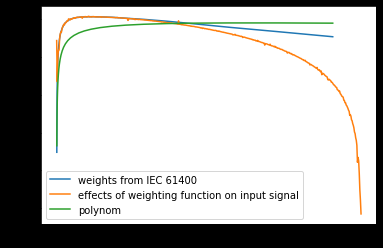I've buid up a polynom that gives exactly the same response as the norm coefficients (note the minus sign on A1000), but still, when i convert it to an analog filter with bilinear, the output is irrelevant :

``````    f1 = 20.598997
f2 = 107.65265
f3 = 737.86223
f4 = 12194.217
A1000 = -2.0

NUMs = [(f4 ** 2), 0, 0, 0, 0]
NUMs = np.polymul(NUMs, NUMs)
DENs = np.polymul([1, 0 , 2 * (f1 ** 2), 0, f1 **4] , [1, 0, f2 ** 2])
DENs = np.polymul(np.polymul(DENs, [1, 0, f3 ** 2]), [1, 0 , 2 * (f4 ** 2), 0, f4 **4])
DENs = DENs * (10**(A1000/10))
``````

Any idea to confirm / correct this?

BR

### endolith commented Oct 8, 2021 • edited

@ThomQu

1. This version is only accurate at high sampling rates, because it's bilinear transformed from the analog filter.
2. You shouldn't use this version anymore, as it says in the readme. The version mentioned in the readme is better at lower sample rates. (Edit: Actually I guess it's not yet. I have a better version with MZTi correction that I haven't pushed yet. I thought I pushed it a long time ago.)
3. I don't understand your graph. Is it linear frequency axis or log?

### ThomQu commented Oct 8, 2021

@endolith

That was quick, thanks!

1. OK for that, but since the frequency-polynom doesn't match with the norm before building-up the filter, it looks that the final error is due to the polynom formula rather than the filtering process, am I right?
2. OK I'm gonna try that other version right away
3. Linear frequencies. Sorry my previous pic was crap, here's the full graph: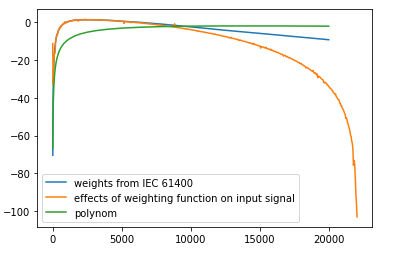### endolith commented Oct 8, 2021

@ThomQu

1. I'm not sure I understand what you're doing. In this version, `ABC_weighting()` produces analog filter coefficients. Then `A_weighting()` converts it to a digital filter using bilinear transform (which has the discrepancy of going to zero at fs/2, so it's only accurate for low frequencies or if your sampling rate is high), then `A_weight()` actually applies that digital filter to a signal.
2. Did you see my edit? The other version isn't actually going to be better at high frequencies, that was a mistake. I need to upload my improved version.
3. So "weights from IEC 61400" is analog frequency response? And "effects of weighting function" is from processing digital white noise? That looks about as I would expect, since the digital filter drops to zero at fs/2. The discrepancy is due to bilinear transform. I don't understand what "polynom" is?

### ThomQu commented Oct 8, 2021 • edited

OK I should have mentioned first that my input signal is sampled at 44 kHz,

The "weights from IEC 61400" curve is just the plotting of the norm's formula: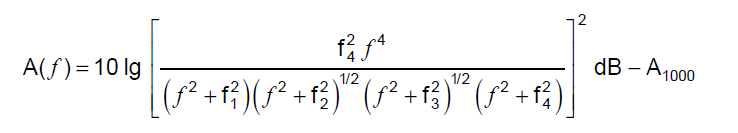i.e. the difference in dB between between an A_weighted spectrum and the same spectrum without weighting

So when I apply the A_weighting function over my white-noise signal, I get a filtered signal in return. I would expect that when I plot its spectrum minus the original spectrum ("effects of weighting function" plot), I would get the same response as the "weights from IEC 61400", at least until 20 kHz (fs/2).

The "polynom" curve was the plotting of NUMs/DENs from this first code's version, applied to the frequencies array and 10-logged:

poly = 10*np.log10(np.polyval(NUMs,f_norm)/np.polyval(DENs,f_norm))
but forget it, I have to dig on filtering's theory (I'm not a spectialist), plotting that and expecting it to be similar to "weights from IEC 61400" might not be relevant...

I've just tested your newer version, the results are indeed equal to this one's.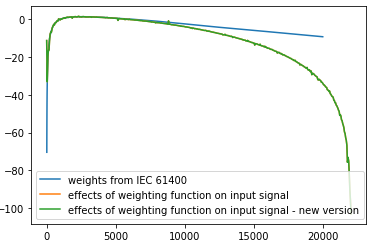### endolith commented Oct 8, 2021 • edited

So I'm looking at my "improved" version from 2018, which is based on the MZTi method and it does indeed have better frequency response at high frequencies: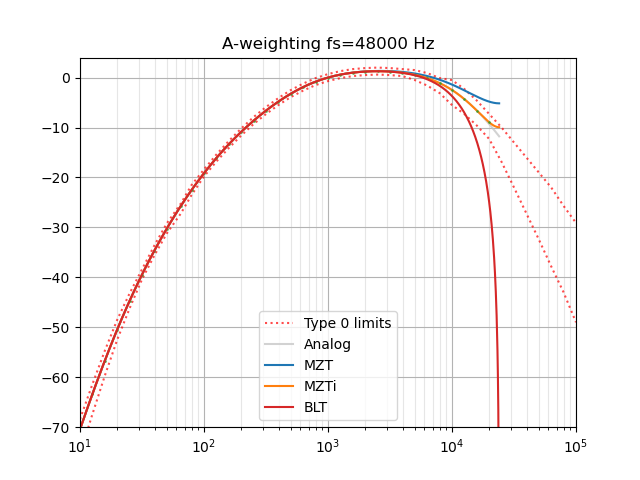But I think the reason I didn't push it because I wasn't sure I was doing it right. "Since the mapping wraps the s-plane's jω axis around the z-plane's unit circle repeatedly, any zeros (or poles) greater than the Nyquist frequency will be mapped to an aliased location." And I'm not sure if those need to be removed first, depending on the sampling rate, etc. At lower sample rates it gets worse, so it still needs some work. (Also the aliased Nyquist tail could be pushed down a bit to improve the overall average accuracy.)

This paper just describes the same BLT solution as this gist, and has a table of the minimum required sample rate to meet the tolerances:

A-weighting C-weighting
IEC 61672-1 Class 1 35 kHz 35 kHz
IEC 61672-1 Class 2 20 kHz 20 kHz
ANSI S.1-43 Type 0 71 kHz 72 kHz
ANSI S.1-43 Type 1 35 kHz 35 kHz
ANSI S.1-43 Type 2 20 kHz 20 kHz

There are probably other papers that describe better methods, I should look for them.

### endolith commented Oct 10, 2021

Can you all tell me how you use this code so I can improve it? I tried to fold it into a library with other related functions like THD+N calculators, etc. But people still comment on this gist, so maybe it's better for it to be self-contained? I assume you are all using the digital filter version and not the analog filter coefficients?

### ThomQu commented Oct 11, 2021

@endolith

Personnaly, I am trying to build-up a library that wraps up a few key functions for quick audio files analyses. I've picked stuff from librosa, scipy, etc and got your code from some colleague's scripts. That's the reason why I went for that first version.

Thks for the info about the MZTi method, I'll look for a little help around to check what I can do with it.

Regards

### endolith commented Oct 13, 2021

@ThomQu

Ok, so maybe I should just make an installable weighting filter library that does nothing else, and then you could use it in yours, etc? I tried combining it with other things like frequency estimation and THD calculation, but maybe those are too unrelated to combine.

### endolith commented Oct 15, 2021 • edited

I was asked on Stack Exchange for my MZTi code, even if crude, so here it is.

I don't think it's correct at very low sample rates, because of aliasing, but should be better at the typical 44.1 or 48 kHz. It's definitely wrong below fs = 2.5 kHz. In between, I think the MZTi zeroes are forcing it into compliance despite being poorly-transformed.

I don't think it makes much practical difference in dB levels vs the BLT method, by the way.

``````# Poles and zeros for analog filter from specification
z, p, k = ABC_weighting('A')

# Matched Z Transform:
T = 1/fs
z_MZT = np.exp(z*T)  # 4
p_MZT = np.exp(p*T)  # 6

k_MZT = k * ((np.prod(2*pi*1000 - z)/
np.prod(2*pi*1000 - p)) *
(np.prod(1 - exp(p*T - 2*pi*1000*T)))/
np.prod(1 - exp(z*T - 2*pi*1000*T)))

# Tweak gain to actual magnitude response TODO: Why is this necessary?
w_MZT, h_MZT = signal.freqz_zpk(z_MZT, p_MZT, k_MZT, [1000/(fs/2)*pi])
k_MZT /= abs(h_MZT)

w = np.array([pi/3, 2*pi/3])
H = abs(signal.freqs_zpk(z, p, k, w*fs) /
signal.freqz_zpk(z_MZT, p_MZT, k_MZT, w))  # relative magnitude error

# MZTi method of 0, 1/3, 2/3 Nyquist will not work, since response at 0 is 0.
H = np.concatenate((, H))

b1 = 0.5 * (H - sqrt(H**2 - 2*H**2 + 2*H**2))
b2 = (3 * (H - b1) -
sqrt(-3*H**2 + 12*H**2 - 6*H*b1 - 3*b1**2 + 0j)
) / 6
b0 = H - b1 - b2
b = np.array([b0, b1, b2])

z_correct = np.roots(b)

# TODO: Is this right?
z_MZTi = np.concatenate((z_MZT, z_correct))
p_MZTi = p_MZT
k_MZTi = k_MZT

# Tweak gain to actual magnitude response again TODO: Why?
w_MZTi, h_MZTi = signal.freqz_zpk(z_MZTi, p_MZTi, k_MZTi, [1000/(fs/2)*pi])
k_MZTi /= abs(h_MZTi)
``````

### endolith commented Oct 14, 2022

so maybe I should just make an installable weighting filter library that does nothing else

and maybe include bilinear as an option so people can compare with the results of other software?